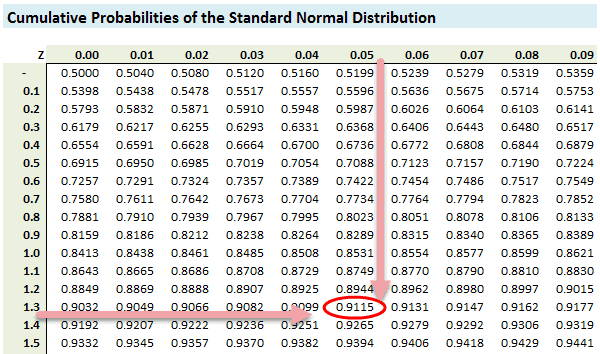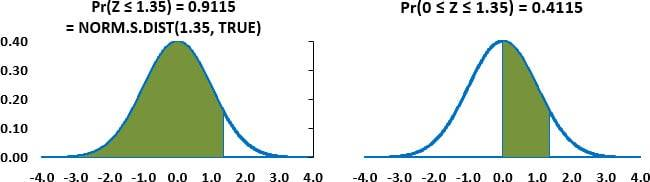# Z Tables

Stay in the know with all things Bionic Turtle

## What Is a Z Table?

A z-table is a mathematical table that allows us to know the percentage of values below a z-score in a standard normal distribution.

## Why Understanding Z-Tables is Important?

Functions based on the normal distribution are easy to retrieve in code or excel, so we do not really need z tables anymore, in practice. But we still want to understand the z table. Why? Because the popular exam calculators (TI BA II+ and HP 12c) do not include z table functionality, so we do need to use them to lookup values on the exam (yes, the z table has been provided in recent FRM® exams). But understanding the z table also helps reinforce a basic grasp of random variables.

## How To Use A Z-Table?

Let’s start with a simple example question which is just my variation on an old FRM exam question:

Assume a random normal variable follows a normal distribution with a mean of 2.30 and a standard deviation of 2.00. What is the probability that this random variable is greater than 5.0?

The same question could be re-phrased into the language of asset returns. Here is same question re-phrased: If an asset’s daily return is normally distributed with mean of 2.30% and daily volatility of 2.00%, what is the probability the asset’s return will be at least 5.0%?

What is Normal Distribution?

I hope you noticed the phrase “normally distributed?” It comes up often in exams. The normal distribution is rarely realistic, but it is popular for learning purposes due to its special properties and what is called parsimony. Parsimony here refers to the normal conveniently has only two parameters, mean and variance. The first step is to standardize the given value of 5.0 into a Z value (aka, Z score):

Z = (5.0 – 2.3)/2.0 = 1.350.

All we’ve done here is translate a normal variable into a standard normal variable. A standard normal variable has zero mean and variance of one (consequently its standard deviation is also one). The Z value of 1.350 means “The value of 5.0 is 1.350 standard deviations above the mean of 2.30.” Now we can use the common Z table to retrieve the associated probability. Below is a typical cumulative Z-value lookup. Because our Z-value is 1.35, we want to go down the rows until we arrive at 1.3, then we want to go across the columns until we arrive at 0.05. That’s because 1.35 = 1.30 + 0.05. We see here that for Z = 1.35, the probability is 0.9115 or 91.15%. Let’s formalize our answer with some notation:

Pr[X ≤ 5.0 | µ(X) = 2.3 and σ(X) = 2.0] = Pr(Z ≤ 1.350) = 91.15%.

Please notice the shift from X to Z. The first function says “The probability that X is less than or equal to 5.0 conditional on a mean of X equal to 2.3 and standard deviation of X equal to 2.0.” The second function, Pr(Z ≤ 1.350), reflects the normalization (translation) from the normal X to the standard normal Z, and we don’t need to specify the mean or standard deviation of the Z.

## Normal Distribution Z Table ChartThe values inside the Z value table are probabilities, so they must lie between 0% and 100% inclusive. What does our 91.15% mean? It is the area under the curve to the left of Z(1.35), see graph on the left:## Z Score Table Example 2

Put another way, and still referring to the plot on the left above, the probability of a standard random normal variable (again, that’s a normal variable with mean of zero and unit standard deviation) resulting in 1.35 or less is about 91.15%, which is the area under the curve to the left of Z(1.35). The total area must be 100% because this is a probability distribution. In terms of our original question then, the probability of our random variable returning less than (or equal to) 5.0 is 91.15% and, consequently, the probability of returning greater than 5.0 is 8.85% = 100% – 91.15%. If we use excel, we get this answer with 8.85% = 1 – NORM.S.DIST(1.35, TRUE) or 8.85% – 1 = NORM.DIST(1.35, 0, 1, TRUE). The later function simply makes explicit the zero mean and unit standard deviation.

The plot on the right above gives the area under the curve that is between zero and 1.35; some Z value tables employ this format instead. Instead of a cumulative probability, the table returns the probability that the standard random normal variable will lie between zero and the critical value. The difference must be 0.50 because half of the area is negative under the curve is negative; in our example, 0.4915 is exactly 0.50 less than 0.9915. How can we quickly identify the difference? We can look at the first entry in the table. For Z = 0, it must be either zero or 0.50.

Now let’s alter the question a bit in order to test our understanding. This is more like an exam question because we need to think a little:

Assume a random normal variable follows a normal distribution with a mean of 2.30 and a standard deviation of 2.0. What is the probability that this random variable falls between zero and 5.0?

This must be less that 91.15% because 91.15% includes all the outcomes below zero. We need to standardize the zero outcome: Z = (0 – 2.3)/2 = -1.150. So we are looking for Pr(-1.150 < Z ≤ 1.35). We already know the probability that Z is less than 1.35 is 91.15%. How do we retrieve Pr(Z ≤ -1.15) from the Z table above which only includes positive Z values? We rely on the natural symmetry of the (standard) normal distribution:

Pr(Z ≤ -1.15) = 1 – Pr(Z ≤ 1.15) = 1 – 0.8749 = 0.1251 or 12.51%; please make sure you understand this step!

We rely on the fact that due to the distribution’s symmetry, 12.51% is both Pr(Z < -1.15) and Pr(Z > 1.15). And we get Pr(Z > 1.15) by subtracting Pr(Z ≤ 1.15) from 100%; Z = +1.15 is a value we can lookup on the table. Now we can answer the full question:

Pr(0 ≤ X ≤ 5.0 | µ = 2.3 and σ = 2.0) = Pr(Z ≤ 1.350) – Pr(Z ≤ -1.15) = 0.9115 – 0.1251 = 78.6%.

Graphically, our probability region excludes the left tail of 12.51% and the right tail of 8.85% which is a total of 21.36% excluded and an included region of 78.64% = 100.0% – 21.36%.

I hope that helps introduce you to the Z table!

## Recent Posts## GARP FRM Pass Rate Information

Professionals who use our services are able to pass the FRM exam at a highly successful rate. Our customers often come to us with little knowledge of how to study for their Global Association of Risk Professionals (GARP) Financial Risk Manager (FRM) certification, yet, they see results after...## Spot Rates

Spot prices are a basic building block in finance, but they are tricky when the commodity is money. When the commodity is money, spot prices are called spot rates (a.k.a., spot interest rate). A spot price is simply the market’s current price to buy or sell a commodity for immediate delivery...## What Is a Z Table?

Functions based on the normal distribution are easy to retrieve in code or excel, so we do not really need z tables anymore, in practice. But we still want to understand the z table. Why? Because the popular exam calculators (TI BA II+ and HP 12c) do not include z table functionality...

### What Your Colleagues Are Saying

I took the exam in Sydney and had a similar feeling about the exam being more qualitative (but no less rigorous). However, I felt I was better prepared thanks to the BT's relentless focus on throwing real life examples and methods.

Jagan G.

I subscribed to BT for my Part 1 FRM exam and just wanted to say thanks for the depth and breadth of the study materials and practice questions. I found out that I scored in the top quartile of every topic and I absolutely could not have done this without using BT - I spent many, many hours going over the practice questions and answers! I wanted to express my appreciation and gratitude to your team for your hard work in creating these materials. Thanks!

Shu C.

The BT scripts, practice questions, global topic drills and mock exams were a great help in understanding the concepts (which I could already apply on the job!) and where structured in such a manner that the breadth and depth where optimal for exam preparation - clearly the exam would have been a catastrophe without BT!

Ivan J.

Passed first time. Happy all the hard work paid off. BT was the right choice. Thanks David and Nicole for your work and commitment.

John D.

Passed! 1,4,1,2,1,4! Thank you David and Nicole for your efforts! Thank you BT! Couldn't have done it otherwise. I'm a mechanical engineer who had a career in petroleum services, then I decided to switch career to financial risk management. Passed part 1 from the first time with top quartiles and passed part 2 from the first time as well. All with BT! BT is always the recommendation I give to people aiming at the FRM designation! Thank you again!

Feras S.

Passed Part I and Part II first time - absolutely could not have done it without BT. Like a few others I didn't even both buying the GARP books for Part II and went solely with BT materials. Just read, answered questions, watched videos, read, more questions, and... more questions! All the practice question taking looked to pay off. Thanks again Bionic Turtle for a great curriculum. Keep up the fantastic work!

John D.